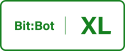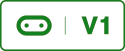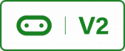# 7. Obstacle Avoidance RobotRequires Ultra Sonic sensor

In this tutorial we will create a obstacle avoidance robot using the HC-SR04 Ultra sonic sensor. This is a cheap sensor that uses sound waves to calculate distances to objects. The function sends out a 10 microsecond pulse, starts a timer then stops the timer when an echo of the pulse is received, Thge round trip time is then used to calculate the distance to the object.

Using Ultrasound to Calculate Distance
``def sonar():  # This function will get distance using the sonar module    global SONAR    start_time = 0    end_time = 0    SONAR.write_digital(1) # Send 10us Ping pulse    sleep_us(10)    SONAR.write_digital(0)    SONAR.set_pull(SONAR.NO_PULL)    while SONAR.read_digital() == 0: # ensure Ping pulse has cleared        pass    start = ticks_us() # define starting time    while SONAR.read_digital() == 1: # wait for Echo pulse to return        pass    end = ticks_us() # define ending time    echo = end-start    distance = int(0.01715 * echo) # Calculate cm distance    return distance``

Now we have a function to detect objects we need to create a simple path finding algorithm so the robot knows how to behave when an object is detected. This will be a simple path finding algorithm that will tell the robot to turn when an object is within 50cm of the sensor. The code below is the comlete code listing where the code under the while loop is the path finding algorithm.

Full Code Listing for a Simple Path Finding Robot
``from microbit import *  # generic microbit functionalityimport neopixel  # used to illuminate the LEDsimport radio  # used to communicate with other microbitsfrom utime import ticks_us, sleep_us, ticks_ms  # used to calc distancerobotType = ""SONAR = pin15minDist = 50  # Distance in CM when the robot will turndef detectModel():  # Detects which model were using XL or classic    global robotType    try:        value = i2c.read(28, 1, repeat=False)  # Read i2c bus        robotType = "XL"  # If we can read it then it must be XL        display.show("X")    except:        robotType = "classic"  # If we can't read it it must be classic        display.show("C")      # or Micro:bit is unplugged    sleep(1000)  # Do this so the user can see if the correct model is founddef sonar():  # This function will get distance using the sonar module    global SONAR    start_time = 0    end_time = 0    SONAR.write_digital(1) # Send 10us Ping pulse    sleep_us(10)    SONAR.write_digital(0)    SONAR.set_pull(SONAR.NO_PULL)    while SONAR.read_digital() == 0: # ensure Ping pulse has cleared        pass    start = ticks_us() # define starting time    while SONAR.read_digital() == 1: # wait for Echo pulse to return        pass    end = ticks_us() # define ending time    echo = end-start    distance = int(0.01715 * echo) # Calculate cm distance    return distancedef Drive(_leftSpeed, _rightSpeed, _leftDirection, _rightDirection):    # speed values between 1 - 1023    # smaller values == faster speed moving backwards    # Smaller values == lower speeds when moving forwards    # direction 0 == forwards, 1 == backwards    leftSpeed.write_analog(_leftSpeed)  # Set the speed of left motor    rightSpeed.write_analog(_rightSpeed)  # Set the speed of right motor    if (_leftDirection != 2):        leftDirection.write_digital(_leftDirection)  # left motor        rightDirection.write_digital(_rightDirection)  # right motor    detectModel()# Motor pins; these tell the motor to go# forward, backwards or turnif robotType == "classic":    leftSpeed = pin0    leftDirection = pin8    rightSpeed = pin1    rightDirection = pin12else:  # Bit:Bot XL    leftSpeed = pin16    leftDirection = pin8    rightSpeed = pin14    rightDirection = pin12    while True:    # path finding alogirthm    distance = sonar()  # get a distance measurement    if distance < minDist:        sleep(200)        Drive(1, 1023, 1,1)    else:        Drive(1023,1023,0,0)            ``

Code Files

pathFinderAUTO.zip